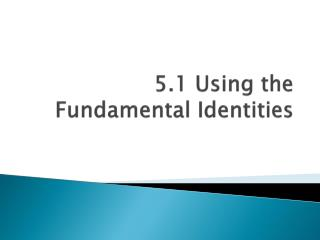# 5.1 Using the Fundamental Identities - PowerPoint PPT PresentationDownload Presentation5.1 Using the Fundamental Identities

5.1 Using the Fundamental IdentitiesDownload Presentation## 5.1 Using the Fundamental Identities

- - - - - - - - - - - - - - - - - - - - - - - - - - - E N D - - - - - - - - - - - - - - - - - - - - - - - - - - -
##### Presentation Transcript

1. 5.1 Using the Fundamental Identities

2. 4 Main Goals: • Evaluate Trig Functions • Simplify Trig Expressions • Develop Additional Trig Identities • Solve Trig Equations

3. Fundamental Trig Identities (page 354) Reciprocal Identities

4. Fundamental Trig Identities (page 354) Quotient Identities

5. Fundamental Trig Identities (page 354) Pythagorean Identities

6. Fundamental Trig Identities (page 354) Cofunction Identities

7. Fundamental Trig Identities (page 354) Even/Odd Identities

8. Using the identities • Use the values and Tan θ > 0 to find the values of all 6 functions.

9. Using the identities • Use the values and Tan θ > 0 to find the values of all 6 functions.

10. Using the identities • Csc x = 4; Cos x < 0

11. Using the identities • Csc x = 4; Cos x < 0

12. Using the Identities • Tan θ is undefined, Sin θ > 0 Tan θ is undefined → Cos θ = 0

13. Using the Identities • Tan θ is undefined, Sin θ > 0 Sin θ = 1 Cos θ = 0 Tan θ is undef. Sec θ = Cot θ = 0 Cscθ = undef. 1

14. Using the identities

15. Simplifying Trig Expressions • To simplify a trig expression means to reduce it to simplest term • This typically means reducing a larger expression to 1 trig function • Never want any fractions in our answer (reciprocal identities)

16. Simplify the following expression:

17. Simplify the following expression:

18. Simplify the following expression:

19. 5.1 Using the Fundamental Identities

23. Match the expressions to one of the following:

24. Keep in mind: • As we continue through the chapter, the problems with increase in difficulty • Always try to use the identities when possible • Last Resort is to convert all to sines and cosines • A common mistake is starting all problems by converting all to sines and cosines. Do this last!

25. 5.1 Using the Fundamental Identities

26. Factoring • So far, all the problems we have done have involved using the identities • Now, your first step should be to look to factor, then try to use the identities • What do you know how to factor?

27. Factoring • Factor out a term Sin x Cos² - Sin x Sin x (Cos²x – 1) • Factor a trinomial Sin²x - 5Sin x + 6 (Sin x – 2) (Sin x – 3) • Factor special polynomials Sin³x - Sin²x – Sin x + 1 (Sin²x – 1) (Sin x - 1)

28. Simplify the following • Sin x Cos²x – Sin x Can we factor? Sin x (Cos²x – 1) Sin x (Sin²x) Sin³ x

29. Simplify the following

30. Simplify the following If you get stuck, let x = Tan x 4x² + x - 3 = (2x + 3) (x – 1)

31. Simplify the following

32. Simplify the following

33. 5.1 Using the Fundamental Identities

34. Trig Substitution • Use the substitution x = 2 Tan θ to express the following expression as a trig function of θ

35. 1. Substitute 2 Tan θ for x 2. Apply the rules for exponents 3. Factor 4. Simplify

36. Simplify the following: • x = 3 Sin θ in the expression • x = 2 Tan θ in the expression • x = 2 Cos θ in the expression